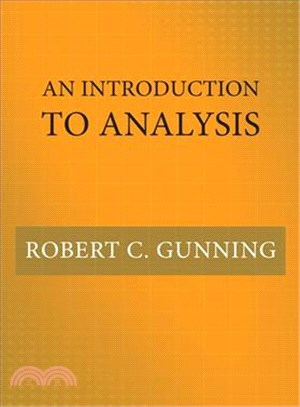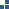瀏覽紀錄

TOP
1/1庫存：1• ## An Introduction to Analysis

• ISBN13：9780691178790
• 出版社：Princeton Univ Pr
• 作者：Robert C. Gunning
• 裝訂／頁數：精裝／376頁
• 規格：25.4cm*17.8cm*3.2cm (高/寬/厚)
• 出版日：2018/03/06杜威分類Natural sciences & mathematics > Analysis
定  價：NT\$3375元
優惠價： 93038
可得紅利積點：91 點

庫存：1

商品簡介

作者簡介

An essential undergraduate textbook on algebra, topology, and calculus

An Introduction to Analysis is an essential primer on basic results in algebra, topology, and calculus for undergraduate students considering advanced degrees in mathematics. Ideal for use in a one-year course, this unique textbook also introduces students to rigorous proofs and formal mathematical writing--skills they need to excel.

With a range of problems throughout, An Introduction to Analysis treats n-dimensional calculus from the beginning—differentiation, the Riemann integral, series, and differential forms and Stokes's theorem—enabling students who are serious about mathematics to progress quickly to more challenging topics. The book discusses basic material on point set topology, such as normed and metric spaces, topological spaces, compact sets, and the Baire category theorem. It covers linear algebra as well, including vector spaces, linear mappings, Jordan normal form, bilinear mappings, and normal mappings.

Proven in the classroom, An Introduction to Analysis is the first textbook to bring these topics together in one easy-to-use and comprehensive volume.

• Provides a rigorous introduction to calculus in one and several variables
• Introduces students to basic topology
• Covers topics in linear algebra, including matrices, determinants, Jordan normal form, and bilinear and normal mappings
• Discusses differential forms and Stokes's theorem in n dimensions
• Also covers the Riemann integral, integrability, improper integrals, and series expansions
Robert C. Gunning is professor of mathematics at Princeton University. His books include Lectures on Riemann Surfaces and Lectures on ComplexAnalytic Varieties (both Princeton).

## 購物須知

為了保護您的權益，「三民網路書店」提供會員七日商品鑑賞期(收到商品為起始日)。

若要辦理退貨，請在商品鑑賞期內寄回，且商品必須是全新狀態與完整包裝(商品、附件、發票、隨貨贈品等)否則恕不接受退貨。

外文館商品之書封，為出版社提供之樣本。實際出貨商品，以出版社所提供之現有版本為主。部份書籍，因出版社供應狀況特殊，匯率將依實際狀況做調整。

無庫存之商品，在您完成訂單程序之後，將以空運的方式為你下單調貨。為了縮短等待的時間，建議您將外文書與其他商品分開下單，以獲得最快的取貨速度，平均調貨時間為1~2個月。